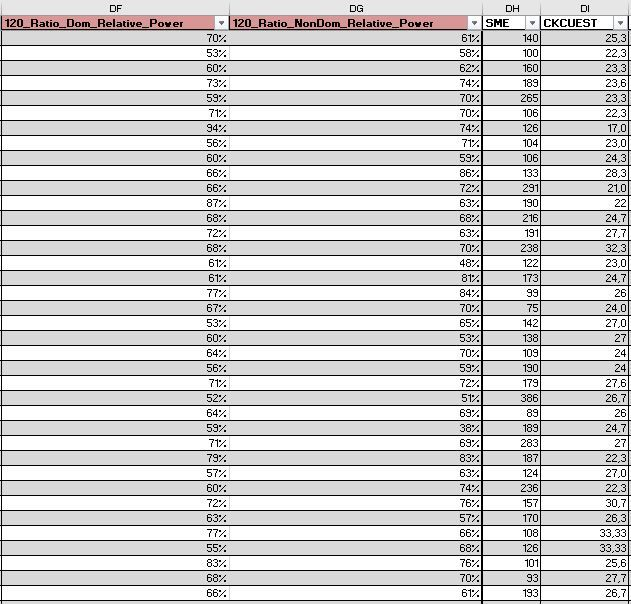Choose Language Hide Translation Bar
Highlighted

## Non-parametric coefficient of determination

Dear,

I have a question about the use of the coefficient of determination (which is part of the output from a regression model).

For the study I'm ready to analyze the data, I want to use the coefficient of determination (COD) to examine the relationship between my dependent variables which represent each column (see annex). But, as I found out each dependent variables (column) has a non consistent distribution of the variance of the residuals.

For this reason I suppose I cannot perform a regression model. But, I wonder what kind of non-parametric test I can use to become some kind of non-parametric coefficient of determination (COD) if this is even possible?Many thanks,

Tomas

2 REPLIES 2
Highlighted

## Re: Non-parametric coefficient of determination

If the anomaly is merely that the residuals from the model are not exhibiting constant variance, then there is an alternative. I assume that your response is continuous numeric data.

Select Analyze > Fit Model. Click the drop-down button next to Personality and select Loglinear Variance. Select the response data column and click Y. Select the factor or predictor data columns. Use the Add, Cross, or Macros buttons to add the model terms to the Mean Effects. Click the Variance Effects tab and repeat the previous step. You are estimating two models: one for the mean of the response and another for the variance of the response. This modeling does not assume that variance is constant with the predicted mean.

Note: the model for the variance is often simpler than the one for the mean. That is, it usually does not exhibit interactions or non-linear effects.

This way does not provide the coefficient of determination, though. The trade-off is losing the COD while gaining the ability to use regression models and learn about the effects on the variance.

Learn it once, use it forever!
Highlighted

## Re: Non-parametric coefficient of determination

Hi,

Many thanks for your response. With this information I can continue my analysis :)

Greetings,
Article Labels

There are no labels assigned to this post.